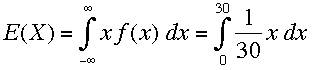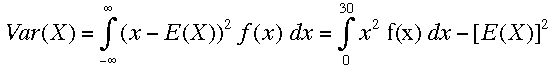SEARCH HOMEMath Central Quandaries & QueriesQuestion from Claudia, a student: A particular employee arrives to work some time between 8:00 am - 8:30 am. Based on past experience the Company has determined that the employee is equally likely to arrive at any time between 8:00 am - 8:30 am. On average, what time does the employee arrive? What is the standard deviation of the time at which the employee arrives? Find the probability that the employee arrives exactly at 8:12 am? Find the probability that the employee arrives between 8:20 am - 8:25 am?Hi Claudia,

I let the random variable X be the time after 8:00 in minutes. Thus 0 ≤ X ≤ 30. Sine the employee is equally likely to arrive at any time X the probability density function f(x) is the uniform distribution so f(x) = 1/30.

The first question is asking for the mean of this distribution, that is the average time the employee arrives after 8:00 isThe standard deviation is the square root of the variance Var(X) whereCan you see how to complete the problem?
HarleyMath Central is supported by the University of Regina and The Pacific Institute for the Mathematical Sciences.